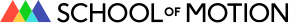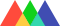#School of Motion## How to Use the Random Expression in After Effects

• By Ryan Plummer
• Share

## Take control of randomly animated movements. Here's how to use the random expression in After Effects.

The random expression in After Effects is both a versatile and essential. There are many applications were the random expression can help ease the burden of small tedious animation tasks that slowly eat at your time animating. However, the random expression can be kinda confusing if you're new to expressions in after effects.
Today I'm going to break down the ins-and-outs of the random expression so you can start incorporating this powerful tool into your motion design workflow. Let's get going and learn how to control the random expression....

## What is the Random Expression in After Effects?

The random expression is used in After Effects to generate random values for the property it's applied to. That seems fairly straight forward, but did you know there are a few different random expressions available in After Effects?
Here's a list of random expressions in that we will cover :
• random(maxValOrArray);
• random(minValOrArray, maxValOrArray);
• gaussRandom(minValOrArray, maxValOrArray);
• seedRandom(seed, timeless = false);
So, you may be asking yourself, "why are there so many options to generate a random number?" Well, As an artist you're going to need a little bit of control, and these various random expressions offer unique ways of controlling randomness in After Effects.
New to Expressions in After Effects?
If you are new to expressions and are wanting to follow along, here is how you add an expression to a property in After Effects:
• Select a layer and find a property you want to affect (like opacity).
• Option (alt on PC) + click on the stopwatch icon next to your desired property.
• Copy and paste, or type the expressions from this article in the expression editor.
Now, let's take a look at the many random expression options...

## Random Expression Breakdown

We're going to go one expression at a time, from easiest to hardest, and breakdown what's happening.
The Basic Random Expression
The most basic form of the random expression in After Effects is simply random();.
`random(50);`
In the example above, After Effects will return random values between 0 and 50 every frame. The number typed in the parentheses is the maximum value we set for this property.
If you were to apply this expression to the opacity layer it would randomly choose a value between 0 and 50 every frame. If you were to type 100 instead of 50, then the opacity value would randomly animate between 0 and 100. Random isn't just for opacity either. You can also randomize just about any property in After Effects, including scale (see below).
Before We Continue...
Something confusing you may run into when working with the random expression is that properties calling for two dimensions, or two values, need special attention. These multi-value 'buckets' are called arrays. It's just a little bit more code to help After Effects interpret what information goes where. For example, some properties that call for two values are scale, position, and anchor point.
```//One value
random(50);
//Two values
p = random(50);
[p,p];```
All we did is place the random expression inside of the variable p, and then typed an array using brackets using the p variable. This will call back the same value for both dimensions.
When you start writing an expression you have to end with the same number of values as you started with. If you're placing an expression on position then you'll start with two numbers, an x and a y value. When wrapping up your expression, After Effects will still be expecting values for both the x and the y position.
When you're working with properties like rotation or opacity you won't have to worry about using an array to wrap up your code. These properties are only using a single value.

### Gaining a Little More Control

If you're looking to add a little bit more control to the range of random values you can type random(minValOrArray, maxValOrArray);.
`random(40,75);`
Okay, so what's happening here and how exactly is this different? In the previous example you only provided one value, which was the maximum allowed value. In this example we are able to set both a minimum and maximum value, allowing us define a custom range of values.
If the expression above was applied to the opacity property, we would generate random numbers between 40 and 75 every frame. You can type any number you want for the minimum and maximum values, but the smaller number should always be the first number.
Interestingly, note that if you typed random(0,100); for opacity this would be the exact same as typing random(100); because 0 is already the minimum value allowed, and 100 is already the maximum value. Pay attention to the property's value limitations when typing out your expressions and this could help you keep your code clean.

### Make your Random Feel Natural

Have you ever heard of Gaussian distribution? Not many people have, and though that may sound complex it's fairly simple to wrap your head around. Let's take a look at our next random expression gaussRandom(); using the opacity property with a range of 0-100%.
Neat image, but how does this apply to this random expression in After Effects? Instead of a uniform distribution of the random values, gaussian distribution will help achieve a more natural look by using a falloff equation. Stay with me here...
From the example above, the values between 40-59% will be chosen approximately 38% of the time. As your values get further from the middle number it will be chosen less. Although this may be obvious, 50% has a slightly higher chance of being picked than 49% due to the falloff of the curve. If this was a raffle between 100 people you would want to be number 50. Your odds of winning would be dramatically better than poor person number 1 or 100!
Here is what gaussRandom looks like in code form:
```gaussRandom(minValOrArray);
gaussRandom(minValOrArray, maxValOrArray);```
Like we explained for the random(); expression above, you can also choose between providing a single value or a range of values for the gaussRandom(); expression.

### How to Control the Random Expression

If you just stick with the random(); expression by itself, what plays back will be different every time. Thankfully the Adobe After Effects team hooked us up with an expression that can help us out.
Random Consistency
Using the seedRandom method will allow you replay the same random movements every time. This is achieved by setting the "seed" value, which will tell After Effects which random algorithm pattern to choose and then use during each playback.
To be clear seedRandom actually won't work by itself. We need to complete this code by using one of the expressions we previously covered. Below I will add in a seed value, and then complete the code by calling the gaussRandom method.
```seedRandom(20, timeless = false);
gaussRandom(20,100);```
Using this small snippet of code you now have a lot more control over your animations. Also, you can exchange gaussRandom(); with random(); to produce a different look.
I should warn you though, the same seed will use the same randomness regardless of the layer it's used on. For example, if you copy and paste an expression from one layer's position to another, they will move in the same exact pattern. To fix this simply change the seed and it will move differently.
True or False
Something that may be confusing about seedRandom(); is the timeless argument. What happens if you change it to true?
It's quite simple and i'll use GIFs to help you wrap your head around how it works. The following GIF has been slowed down with an expression posterizeTime();
If you leave the timeless argument equal to false your value will change each frame. In the example below the Timeless set to "true".
Setting it "true" makes the seed "timeless" which means it stays the same on each frame, but it will still choose a random value.
The opacity value could randomly set to 50%, and then it would remain that way throughout playback. Simply change the seed value if you want a different opacity value.
Pro Tip: Use the Layer Index with the Random Expression
Setting a specific seed value every time you implement this code can be tedious. Here's a simple solution that will lift some of that burden and help save you time.
Instead of typing in a numerical value, you can have After Effects use the layers number.
If you use index in your expression for layer 20, then the seed number will be 20. Doing this will make sure that changes in value won't follow the same patterns of other layers in your composition. To accomplish this simply type the word index where the seed value goes, like so:
```seedRandom(index, false);
gaussRandom(20,100);```
This is extremely helpful if you are copying and pasting expressions across several layers, and will save you time from going into each layer and changing the seed.

## A Cool Example For the Random Expression

Let's take some of that knowledge we just learned and show you something cool! Here's a rig that randomly offset when a layer should animate on.
We used the random expression in this example to let After Effects offset and choose when our animation should start! What's great is that it is random for every layer. Could you imagine having more circles than that? Without the random expression you'd have to animate each one of them differently or even offset each layer. That would take a really long time!
The example above utilizes a little more expression knowledge than we explained here, so we're going to give away the project file for free! And, since there is a little bit more expression magic happening in this file I sprinkled in a few notes to help you reverse engineer the project.